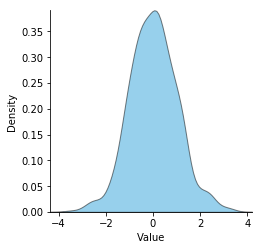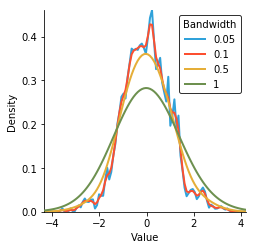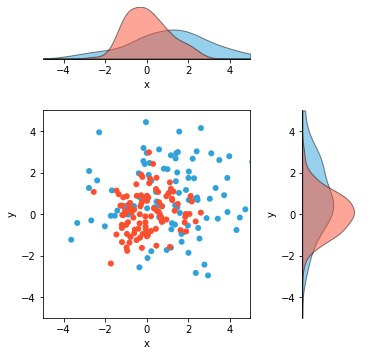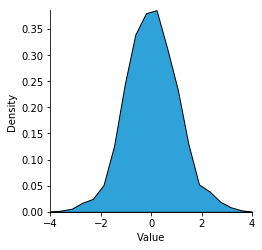# Distribution¶

Title
Distribution Element
Dependencies
Matplotlib, SciPy
Backends
Matplotlib
Bokeh
Plotly
In :
import numpy as np
import holoviews as hv
hv.extension('matplotlib')Distribution provides a convenient way to visualize a 1D distribution of values as a Kernel density estimate. Kernel density estimation is a non-parametric way to estimate the probability density function of a random variable.

The KDE works by placing a Gaussian kernel at each sample with the supplied bandwidth, which are then summed to produce the density estimate. By default the bandwidth is determined using the Scott's method, which usually produces good results, but it may be overridden by an explicit value.

To start with we will create a Distribution with 1,000 normally distributed samples:

In :
normal = np.random.randn(1000)
hv.Distribution(normal)

Out:We can set explicit values for the bandwidth to see the effect and also declare whether we want the plot to be filled:

In :
hv.NdOverlay({bw: hv.Distribution(normal).opts(alpha=1, bandwidth=bw, filled=False)
for bw in [0.05, 0.1, 0.5, 1]}, 'Bandwidth')

Out:The Distribution element is also useful to visualize the marginal distribution of a set of points. Here we will declare distributions for the x- and y-values of two sets of Points with slightly different spreads and means and then adjoin these plots:

In :
points = hv.Points(np.random.randn(100,2))
points2 = hv.Points(np.random.randn(100,2)*2+1)

xdist, ydist = ((hv.Distribution(points2, kdims=[dim]) *
hv.Distribution(points, kdims=[dim]))
for dim in 'xy')
((points2 * points) << ydist << xdist).redim.range(x=(-5, 5), y=(-5, 5))

Out:Underlying the Distribution element is the univariate_kde operation, which computes the KDE for us automatically when we plot the element. We can also use this operation directly and print the output highlighting the fact that the operation simply returns an Area or Curve element. It also affords more control over the parameters letting us directly set not only the bandwidth and cut values but also a bin_range, bw_method and the number of samples (n_samples) to approximate the KDE with:

In :
from holoviews.operation.stats import univariate_kde
dist = hv.Distribution(normal)
kde = univariate_kde(dist, bin_range=(-4, 4), bw_method='silverman', n_samples=20)
kde

Out:For full documentation and the available style and plot options, use hv.help(hv.Distribution).